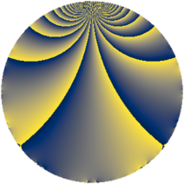# Properties

 Label 819.2.bmLevel $819$ Weight $2$ Character orbit 819.bm Rep. character $\chi_{819}(478,\cdot)$ Character field $\Q(\zeta_{6})$ Dimension $90$ Newform subspaces $8$ Sturm bound $224$ Trace bound $5$

# Related objects

## Defining parameters

 Level: $$N$$ $$=$$ $$819 = 3^{2} \cdot 7 \cdot 13$$ Weight: $$k$$ $$=$$ $$2$$ Character orbit: $$[\chi]$$ $$=$$ 819.bm (of order $$6$$ and degree $$2$$) Character conductor: $$\operatorname{cond}(\chi)$$ $$=$$ $$91$$ Character field: $$\Q(\zeta_{6})$$ Newform subspaces: $$8$$ Sturm bound: $$224$$ Trace bound: $$5$$ Distinguishing $$T_p$$: $$2$$, $$5$$

## Dimensions

The following table gives the dimensions of various subspaces of $$M_{2}(819, [\chi])$$.

Total New Old
Modular forms 240 98 142
Cusp forms 208 90 118
Eisenstein series 32 8 24

## Trace form

 $$90q - 90q^{4} + q^{7} + O(q^{10})$$ $$90q - 90q^{4} + q^{7} + 9q^{10} + 9q^{11} - 8q^{13} + 14q^{14} + 94q^{16} + 14q^{17} - 12q^{19} + 30q^{20} + 6q^{22} + 14q^{23} + 35q^{25} + 20q^{26} + 11q^{28} + 4q^{29} + 9q^{31} + 11q^{35} - 32q^{38} - 24q^{40} - 3q^{41} + 12q^{44} - 36q^{47} + 11q^{49} + 78q^{50} + 63q^{52} + 7q^{53} - 16q^{55} - 27q^{56} + 57q^{58} - 40q^{61} + 2q^{62} - 104q^{64} - 14q^{65} + 12q^{67} - 26q^{68} - 18q^{70} - 51q^{71} + 9q^{73} - 42q^{74} + 30q^{76} - 48q^{77} + 16q^{79} + 30q^{80} + 20q^{82} - 51q^{85} + 54q^{86} - 5q^{88} + 9q^{91} - 74q^{92} - 18q^{94} + 50q^{95} - 30q^{97} - 60q^{98} + O(q^{100})$$

## Decomposition of $$S_{2}^{\mathrm{new}}(819, [\chi])$$ into newform subspaces

Label Dim. $$A$$ Field CM Traces $q$-expansion
$$a_2$$ $$a_3$$ $$a_5$$ $$a_7$$
819.2.bm.a $$2$$ $$6.540$$ $$\Q(\sqrt{-3})$$ None $$0$$ $$0$$ $$-3$$ $$-4$$ $$q+(-1+2\zeta_{6})q^{2}-q^{4}+(-2+\zeta_{6})q^{5}+\cdots$$
819.2.bm.b $$2$$ $$6.540$$ $$\Q(\sqrt{-3})$$ None $$0$$ $$0$$ $$6$$ $$5$$ $$q+(1-2\zeta_{6})q^{2}-q^{4}+(4-2\zeta_{6})q^{5}+\cdots$$
819.2.bm.c $$2$$ $$6.540$$ $$\Q(\sqrt{-3})$$ $$\Q(\sqrt{-3})$$ $$0$$ $$0$$ $$0$$ $$-4$$ $$q+2q^{4}+(-3+2\zeta_{6})q^{7}+(-3+4\zeta_{6})q^{13}+\cdots$$
819.2.bm.d $$4$$ $$6.540$$ $$\Q(\sqrt{-3}, \sqrt{-7})$$ None $$0$$ $$0$$ $$-6$$ $$0$$ $$q+(\beta _{1}-\beta _{3})q^{2}+(-1+\beta _{1}+\beta _{3})q^{4}+\cdots$$
819.2.bm.e $$12$$ $$6.540$$ 12.0.$$\cdots$$.1 None $$0$$ $$0$$ $$6$$ $$-3$$ $$q+(\beta _{1}+\beta _{3}+\beta _{6})q^{2}+(-\beta _{1}+\beta _{2}+\beta _{3}+\cdots)q^{4}+\cdots$$
819.2.bm.f $$12$$ $$6.540$$ 12.0.$$\cdots$$.1 None $$0$$ $$0$$ $$3$$ $$-3$$ $$q+\beta _{9}q^{2}+(-1-\beta _{2}-\beta _{4}+\beta _{5}+\beta _{7}+\cdots)q^{4}+\cdots$$
819.2.bm.g $$20$$ $$6.540$$ $$\mathbb{Q}[x]/(x^{20} + \cdots)$$ None $$0$$ $$0$$ $$-6$$ $$2$$ $$q+(\beta _{2}+\beta _{3})q^{2}+(-1+\beta _{1})q^{4}+\beta _{7}q^{5}+\cdots$$
819.2.bm.h $$36$$ $$6.540$$ None $$0$$ $$0$$ $$0$$ $$8$$

## Decomposition of $$S_{2}^{\mathrm{old}}(819, [\chi])$$ into lower level spaces

$$S_{2}^{\mathrm{old}}(819, [\chi]) \cong$$ $$S_{2}^{\mathrm{new}}(91, [\chi])$$$$^{\oplus 3}$$$$\oplus$$$$S_{2}^{\mathrm{new}}(273, [\chi])$$$$^{\oplus 2}$$